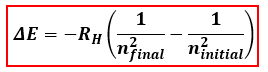# Problem: A hydrogen atom absorbs a photon with a wavelength of 397.1 nm, which excites the atom’s electron. Determine the electron’s initial quantum level if the transition results in a final quantum level of n = 7.a) n = 4b) n = 3c) n = 1d) n = 2e) n = 5

🤓 Based on our data, we think this question is relevant for Professor Andino Martinez's class at UIUC.

###### FREE Expert Solution

We can determine initial quantum level or initial energy level (ninitial) of the electron using the Bohr Equation shown below:ΔE = energy related to the transition, J/atom
RH = Rydberg constant, 2.178x10-18 J
ni = initial principal energy level
nf = final principal energy level

The energy is not given. However, we can calculate it using the wavelength of the absorbed photon and the equation for the energy of a photon.###### Problem Details

A hydrogen atom absorbs a photon with a wavelength of 397.1 nm, which excites the atom’s electron. Determine the electron’s initial quantum level if the transition results in a final quantum level of n = 7.

a) n = 4

b) n = 3

c) n = 1

d) n = 2

e) n = 5